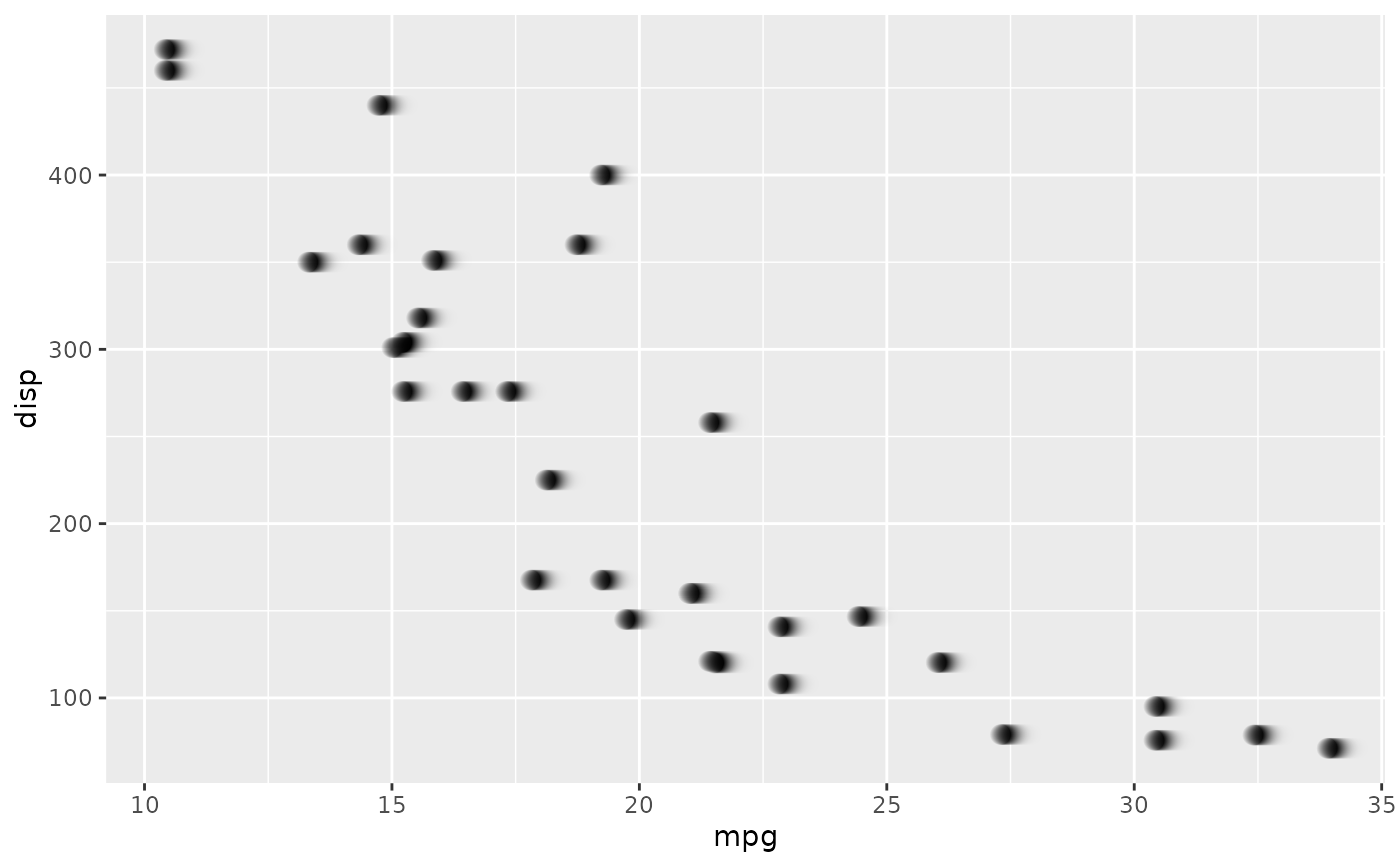This filter allows you to apply a custom kernel to your layer, thus giving you more control than e.g. with_blur() which is also applying a kernel.

with_kernel(
x,
kernel = "Gaussian:0x2",
iterations = 1,
scaling = NULL,
bias = NULL,
stack = FALSE,
...
)

## Arguments

x

A ggplot2 layer object, a ggplot, a grob, or a character string naming a filter

kernel

either a square matrix or a string. The string can either be a parameterized kerneltype such as: "DoG:0,0,2" or "Diamond" or it can contain a custom matrix (see examples)

iterations

number of iterations

scaling

string with kernel scaling. The special flag "!" automatically scales to full dynamic range, for example: "50%!"

bias

output bias string, for example "50%"

stack

Should the original layer be placed on top?

...

Arguments to be passed on to methods. See the documentation of supported object for a description of object specific arguments.

## Value

Depending on the input, either a grob, Layer, list of Layers, guide, or element object. Assume the output can be used in the same context as the input.

## Examples

library(ggplot2)
# Add directional blur using the comet kernel
ggplot(mtcars, aes(mpg, disp)) +
with_kernel(geom_point(size = 3), 'Comet:0,10')